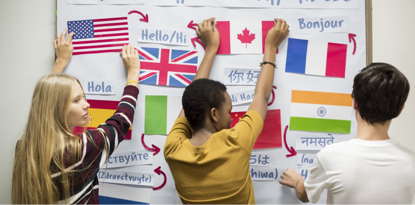# January 2018 - Beverly Hills, CA

ACADEMIC CREDENTIALS EVALUATION INSTITUTE, INC. (ACEI)
Is incorporated in the state of California (1994), and is dedicated to the advancement of international academic exchange and understanding through the dissemination of information on international academic credentials.

 table div table+table+table div table{width:100%;padding:0}table div table+table+table div table img{width:96.23%;padding:0;float:none}table div table+table+table div table td{width:100%;padding:0 1.88% 18px}/* styles */Photo: Embassy of Canada to Vietnam

# International Student Numbers On the Rise

The number of international students seeking a Canadian education continues to grow sharply, with Canada’s immigration division Immigration, Refugees and Citizenship Canada (IRCC) revealing a 22% increase in students who entered Canada last year.

 table div table+table+table+table+table div table{width:100%;padding:0}table div table+table+table+table+table div table img{width:96.23%;padding:0;float:none}table div table+table+table+table+table div table td{width:100%;padding:0 1.88% 18px}/* styles */## CHINA

According to a recent report from the Ministry of Education, China ranks first in the number of Massive Online Open Courses－with 3,200 launched by 460 higher education institutes, including 200 courses available on foreign MOOC platforms.

 table div table+table+table+table+table+table+table div table{width:100%;padding:0}table div table+table+table+table+table+table+table div table img{width:96.23%;padding:0;float:none}table div table+table+table+table+table+table+table div table td{width:100%;padding:0 1.88% 18px}/* styles */# India Allows 15% of Universities to Offer Online Degrees

For the first time, India will allow nearly 15% of universities to offer online degrees allowing students and executives to learn anywhere, anytime.

 table div table+table+table+table+table+table+table+table+table div table{width:100%;padding:0}table div table+table+table+table+table+table+table+table+table div table img{width:96.23%;padding:0;float:none}table div table+table+table+table+table+table+table+table+table div table td{width:100%;padding:0 1.88% 18px}/* styles */# Universities Scramble to Select 70,000 Students

Panic has hit universities as they scramble to admit 70,000 students who scored the minimum entry grade of C+ starts next week.

 table div table+table+table+table+table+table+table+table+table+table+table+table div table{width:100%;padding:0}table div table+table+table+table+table+table+table+table+table+table+table+table div table img{width:96.23%;padding:0;float:none}table div table+table+table+table+table+table+table+table+table+table+table+table div table td{width:100%;padding:0 1.88% 18px}/* styles */# Education Ministry Blasts Universities as Sub-par

The Office of Higher Education Commission (Ohec) of Thailand recently reported that almost 200 university curricula have fallen short of the required standards, adding it would name them on its website shortly.

 table div table+table+table+table+table+table+table+table+table+table+table+table+table+table+table div table{width:100%;padding:0}table div table+table+table+table+table+table+table+table+table+table+table+table+table+table+table div table img{width:96.23%;padding:0;float:none}table div table+table+table+table+table+table+table+table+table+table+table+table+table+table+table div table td{width:100%;padding:0 1.88% 18px}/* styles */# Scrapping of the Assistant Professor Titles

A series of radical changes to Turkey’s university system being brought by the Justice and Development Party (AKP) to the Turkish parliament include the scrapping of the assistant professor role, the abolition of the oral exam and the lowering of foreign language conditions required of academics seeking promotion to associate professor, and a greater role for universities in the hiring of staff.

 table div table+table+table+table+table+table+table+table+table+table+table+table+table+table+table+table+table+table div table{width:100%;padding:0}table div table+table+table+table+table+table+table+table+table+table+table+table+table+table+table+table+table+table div table img{width:96.23%;padding:0;float:none}table div table+table+table+table+table+table+table+table+table+table+table+table+table+table+table+table+table+table div table td{width:100%;padding:0 1.88% 18px}/* styles */# Diploma Mills: Degrees of Deception

Listen to the BBC Radio 4 report on diploma mills and AXACT, the Karachi, Pakistan-based diploma mill that was shut down by the authorities after an NYT expose but is back in business. For a transcript of this report, click here. You can listen to the audio, click here

 table div table+table+table+table+table+table+table+table+table+table+table+table+table+table+table+table+table+table+table+table+table div table{width:100%;padding:0}table div table+table+table+table+table+table+table+table+table+table+table+table+table+table+table+table+table+table+table+table+table div table img{width:96.23%;padding:0;float:none}table div table+table+table+table+table+table+table+table+table+table+table+table+table+table+table+table+table+table+table+table+table div table td{width:100%;padding:0 1.88% 18px}/* styles */# International Student Numbers in US on the Decline

According to a new report released this month from the National Science Foundation, the number of international students in the U.S. fell by 2.2 percent at the undergraduate level and 5.5 percent at the graduate level from fall 2016 to 2017.

 table div table+table+table+table+table+table+table+table+table+table+table+table+table+table+table+table+table+table+table+table+table+table+table+table div table{width:100%;padding:0}table div table+table+table+table+table+table+table+table+table+table+table+table+table+table+table+table+table+table+table+table+table+table+table+table div table img{width:96.23%;padding:0;float:none}table div table+table+table+table+table+table+table+table+table+table+table+table+table+table+table+table+table+table+table+table+table+table+table+table div table td{width:100%;padding:0 1.88% 18px}/* styles */Image by Joanne Cheung

# Exploring Climate Change through Art and Design

Harvard University Graduate student, Joanne Cheung, explores perception of climate change through design and art.

 table div table+table+table+table+table+table+table+table+table+table+table+table+table+table+table+table+table+table+table+table+table+table+table+table+table+table+table div table{width:100%;padding:0}table div table+table+table+table+table+table+table+table+table+table+table+table+table+table+table+table+table+table+table+table+table+table+table+table+table+table+table div table img{width:96.23%;padding:0;float:none}table div table+table+table+table+table+table+table+table+table+table+table+table+table+table+table+table+table+table+table+table+table+table+table+table+table+table+table div table td{width:100%;padding:0 1.88% 18px}/* styles */# Exploring the World Through Music

To guide us on this musical journey, ACEI guest blogger and music aficionado, Tom Schnabel, shares with us a list of his favorites from around the globe.

 table div table+table+table+table+table+table+table+table+table+table+table+table+table+table+table+table+table+table+table+table+table+table+table+table+table+table+table+table+table div table{width:100%;padding:0}table div table+table+table+table+table+table+table+table+table+table+table+table+table+table+table+table+table+table+table+table+table+table+table+table+table+table+table+table+table div table img{width:96.23%;padding:0;float:none}table div table+table+table+table+table+table+table+table+table+table+table+table+table+table+table+table+table+table+table+table+table+table+table+table+table+table+table+table+table div table td{width:100%;padding:0 1.88% 18px}/* styles */# The End of Foreign Language Education in US Schools

ACEI’s Frustrated Evaluator shares his exasperation with the absence of foreign language instruction at US schools.

 table div table+table+table+table+table+table+table+table+table+table+table+table+table+table+table+table+table+table+table+table+table+table+table+table+table+table+table+table+table+table+table div table{width:100%;padding:0}table div table+table+table+table+table+table+table+table+table+table+table+table+table+table+table+table+table+table+table+table+table+table+table+table+table+table+table+table+table+table+table div table img{width:96.23%;padding:0;float:none}table div table+table+table+table+table+table+table+table+table+table+table+table+table+table+table+table+table+table+table+table+table+table+table+table+table+table+table+table+table+table+table div table td{width:100%;padding:0 1.88% 18px}/* styles */